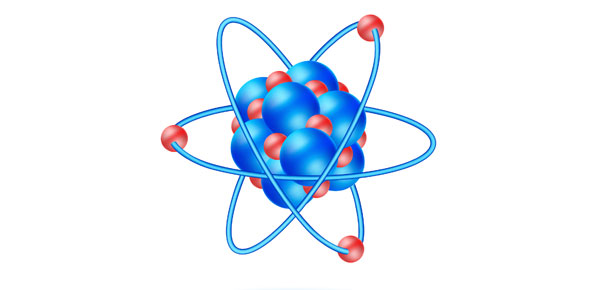# Base Quantities And Derived Quantities

4 Questions | Total Attempts: 1542SettingsA physical quantity is anything that you can measure. For example, length, temperature,distance and time are physical quantities.

Related Topics
• 1.
Which of the following is a base quantity?
• A.

Weight

• B.

Energy

• C.

Mass

• D.

Velocity

• 2.
The SI units for length, time and mass are respectively
• A.

Metre , second and gram

• B.

Metre , second and kilogram

• C.

Centimetre, minute and gram

• D.

Centimetre, hour and kilogram

• 3.
Which one of the following lists consists of three base quantities?
• A.

Electric current, length and area

• B.

Mass, time and volume

• C.

Distance, displacement and volume

• D.

Length, temperature and electric current

• 4.
The symbol for mass, electric current and time are respectively;
• A.

M,I and t

• B.

Kg, A and s

• C.

M, I and T

• D.

I, A and t